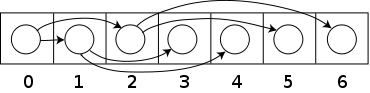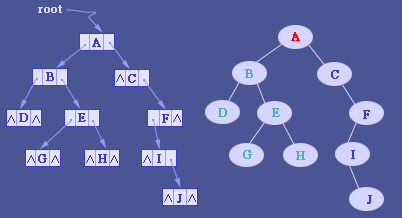# 数据结构之二叉树递归操作

1.每个结点最多有两棵字树，所以二叉树中不存在度大于2的结点
2.二叉树是有序的，其次序不能任意颠倒，即使树中的某个结点只有一棵

1.斜树2.满二叉树3.完全二叉树

1.如果有度为1的结点，只可能有一个，且该结点只有左孩子。
2.叶子结点只能出现在最下面两层，且最下层的叶子结点都集中在二叉树左侧连续的位置。1. #include<iostream>
2. using namespace std;
3. typedef struct node
4. {
5.     struct node *leftChild;
6.     struct node *rightChild;
7.     char data;
8. }BiTreeNode, *BiTree;

9. void createBiTree(BiTree &);
10. void PreOrderBiTree(BiTree *);
11. void InOrderBiTree(BiTree *);
12. void PostOrderBiTree(BiTree *);
13. int BiTreeDeep(BiTree *);
14. int LeafTreecount(BiTree *);

15. void createBiTree(BiTree &T)
16. {
17.     char c;
18.     cin >> c;
19.     if ('#' == c)
20.         T = NULL;
21.     else
22.     {
23.         T = new BiTreeNode;
24.         T->data = c;
25.         createBiTree(T->leftChild);
26.         createBiTree(T->rightChild);
27.     }
28. }

29. void PreOrderBiTree(BiTree &T)
30. {
31.     if (T == NULL)
32.         return;
33.     cout << T->data << " ";
34.     PreOrderBiTree(T->leftChild);
35.     PreOrderBiTree(T->rightChild);

36. }
37. void InOrderBiTree(BiTree &T) {

38.     if (T == NULL)
39.         return;
40.         InOrderBiTree(T->leftChild);
41.         cout << T->data << " ";
42.         InOrderBiTree(T->rightChild);
43.
44. }
45. void PostOrderBiTree(BiTree &T) {
46.     if (T == NULL)
47.         return;
48.     PostOrderBiTree(T->leftChild);
49.     PostOrderBiTree(T->rightChild);
50.     cout << T->data<<" ";
51. }

52. int BiTreeDeep(BiTree T)
53. {
54.     int deep = 0;
55.     if (T)
56.     {
57.         int leftdeep = BiTreeDeep(T->leftChild);
58.         int rightdeep = BiTreeDeep(T->rightChild);
59.         deep = leftdeep >= rightdeep ? leftdeep + 1 : rightdeep + 1;
60.     }
61.     return deep;
62. }

63. //求二叉树叶子结点个数

64. int LeafTreecount(BiTree T,int &num)
65. {
66.     if (T)
67.     {
68.         if (T->leftChild == NULL &&T->rightChild == NULL)
69.             num++;
70.         LeafTreecount(T->leftChild,num);
71.         LeafTreecount(T->rightChild,num);

72.     }
73.     return num;
74. }
75. void DeleteBiTree(BiTree T) {
76.     if (T == NULL)
77.         return;
78.     DeleteBiTree(T->leftChild);
79.     DeleteBiTree(T->rightChild);
80.     delete T;
81.
82. }
83. int main()
84. {
85. BiTree T;
86. cout << "输入的字符是：" << endl;
87. createBiTree(T);
88. cout << "前序遍历:" << endl;
89. PreOrderBiTree(T); \
90. cout << endl;
91. cout <<"中序遍历:"<< endl;
92. InOrderBiTree(T);
93. cout << endl;
94. cout << "后序遍历:" << endl; \
95. PostOrderBiTree(T);
96. cout << endl;
97. cout << "二叉树的高度为：" << BiTreeDeep(T) << endl;
98. int num = 0;
99. cout << "二叉树的叶子结点个数为:" << LeafTreecount(T, num)<< endl;
100. DeleteBiTree(T);
101. cout << "树已销毁！！！" << endl;
102. return 0;
103. }• 博文量
159
• 访问量
988866